Next jam: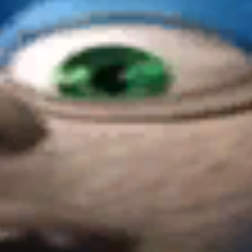Stolen math by godde What if you could just take a game from Unity Store and upload it?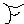Fractals by samurai_worf Click the Button to add one Iteration ( up to 13 ) to the Fractal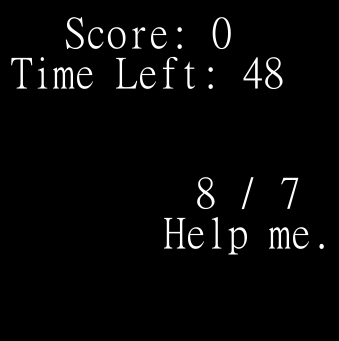MATH MINUTES by 👁 qst0 👁 DO MATH! Don't worry about remainders for dividing, that's too hard right? In example: 7 / 4 = 1 Also, that angle game might be a bit strange. Have... fun?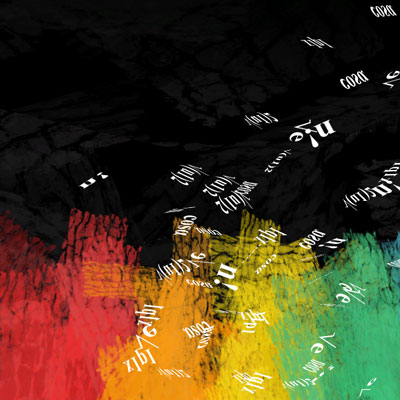A Problem by wan All problems can be solved with the right answer.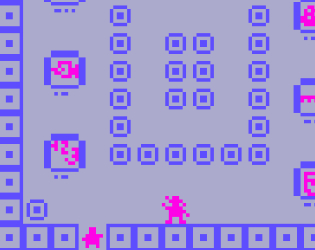Math Museum by happyturtle5 Educational game about math.Math except it isn't math by admiral_octavio You see, the Maths in this game is actually in the code and textures of it. You can press arrow keys to rotate everything and Z and X for zooming :D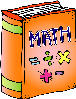π by dmoa left and right arrows to move. first web game in over a year!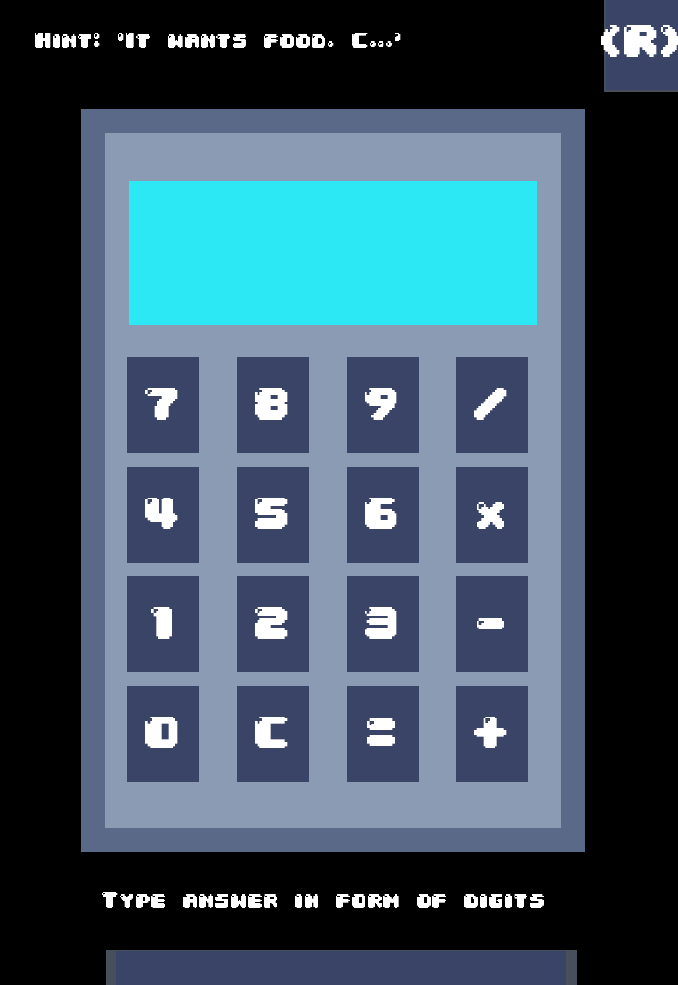Answer is puzzle by ulcius You need to type answer to the puzzle in the form of right digits. What are right digits? Well... 0 means A. Wonder, where you can find B,C,D etc? Try find pattern and make calculations Click '=' after calculations to see if you found the pattern and the letter under it :)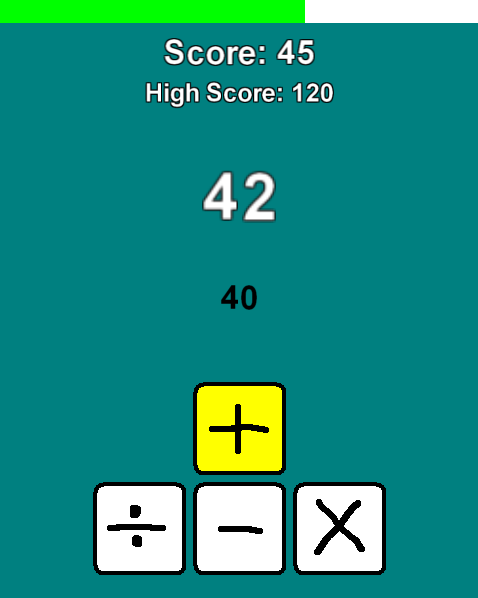Reaching the Number by tirnam Just reaching the number